# Rational numbers - math word problems

#### Number of problems found: 168

• FractionFraction ? write as fraction a/b, a, b is integers numerator/denominator.
• Bureaucracy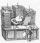The office was attended by 23 people. 9 of them completed the questionnaire. What is the percentage of all people?
• Permill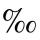How many permill is 978 from 84370?
• What is 11What is the quotient of Three-fifths and 1 Over 10?
• Ratio - proportion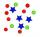Reduce the number 16 in proportion 3:2 5:4 11:8
• Lowest termsReduce to the lowest terms: 32/124
• Decimal to fractionWrite decimal number 8.638333333 as a fraction A/B in the basic form. Given decimal has infinite repeating figures.
• A numberA number increased by 7.9 is 8.3
• Find the 21Find the sum of the six terms of the finite geometric sequence 96, -48, 24, -12
• Package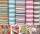The package was 23 meters of textile. The first day sold 12.3 meters. How many meters of textile remained in the package?
• What is one third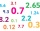What is 1/3 as a decimal? Give your answer rounded to 2 decimal places.
• Insert 3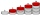Insert five arithmetic progression members between -7 and 3/2.
• Division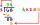Divide by the number 0.2 is the same as multiply by what number?
• Car inflation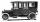About what percentage price of car increased if price rose from €1880 to €2200?
• Dinesh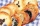Dinesh ate 3 cookies, each with mass 1.45 g. What was the total mass of the cookies that he ate?
• Rounding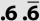Double round number 727, first to tens, then to hundreds. (double rounding)
• DayWhat part of the day is 23 hours 22 minutes? Express as a decimal number.
• Percent to number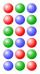To increase that number by 5 percent, we need to multiply it by:
• Comparing powers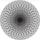How many times is number 56 larger than number 46?
• Efficiency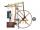A certain device consumes 28 kW and delivers 14 kW. Find its efficiency.

Do you have an interesting mathematical word problem that you can't solve it? Submit a math problem, and we can try to solve it.

We will send a solution to your e-mail address. Solved examples are also published here. Please enter the e-mail correctly and check whether you don't have a full mailbox.

Please do not submit problems from current active competitions such as Mathematical Olympiad, correspondence seminars etc...